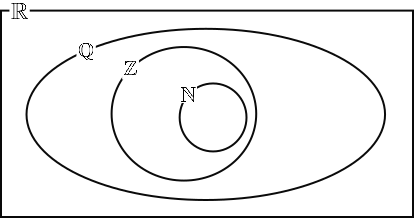# Exam-Style Questions on Number

## Problems on Number adapted from questions set in previous Mathematics exams.

### 1.

GCSE Higher

Find the highest common factor (HCF) of 60 and 96.

### 2.

GCSE Higher

Without using a calculator:

(a) Express 68 as the product of its prime factors.

(c) Multiply 13.06 by 2.1

### 3.

GCSE Higher

The number, $$N$$, can be written as the product of prime factors in index form as:

$$N = 3 × 5^3 × x^4$$

Work out $$5N^2$$ as a product of prime factors in index form giving your answer in terms of $$x$$.

### 4.

GCSE Higher

The difference between the areas of the two squares is 51 cm2.

When measured in cm the lengths of the sides of the squares are integers and both less than 20cm.

Find the lengths of the sides of the two squares.

### 5.

IB Studies

This Venn diagram shows the relationship between the sets of numbers

$$\mathbb N, \mathbb Z, \mathbb Q \text{ and } \mathbb R$$Write down the following numbers in the appropriate place in the Venn diagram.

(a) $$5$$

(b) $$\frac23$$

(c) $$\pi$$

(d) $$0.91$$

(e) $$\sqrt 7$$

(f) $$-0.75$$

### 6.

GCSE Higher

This expression can be used to generate a sequence of numbers.

$$n^2+n + 5$$

(a) Work out the first three terms of this sequence.

(b) What is the smallest value of $$n$$ that produces a term of the sequence that is not a prime number?

(c) Is it true that odd square numbers have exactly three factors? Explain and justify your answer.

(d) Seymour is thinking of a number.

• It is a common factor of 144 and 192.
• It is a common multiple of 6 and 8.
• It is less than 100.

Find the two possible numbers that Seymour could be thinking of.

If you would like space on the right of the question to write out the solution try this Thinning Feature. It will collapse the text into the left half of your screen but large diagrams will remain unchanged.

The exam-style questions appearing on this site are based on those set in previous examinations (or sample assessment papers for future examinations) by the major examination boards. The wording, diagrams and figures used in these questions have been changed from the originals so that students can have fresh, relevant problem solving practice even if they have previously worked through the related exam paper.

The solutions to the questions on this website are only available to those who have a Transum Subscription.

Exam-Style Questions Main Page

Search for exam-style questions containing a particular word or phrase:

To search the entire Transum website use the search box in the grey area below.